Research papers and code for "Kevin Jamieson":
This paper establishes that optimistic algorithms attain gap-dependent and non-asymptotic logarithmic regret for episodic MDPs. In contrast to prior work, our bounds do not suffer a dependence on diameter-like quantities or ergodicity, and smoothly interpolate between the gap dependent logarithmic-regret, and the $\widetilde{\mathcal{O}}(\sqrt{HSAT})$-minimax rate. The key technique in our analysis is a novel "clipped" regret decomposition which applies to a broad family of recent optimistic algorithms for episodic MDPs.

Click to Read Paper and Get Code
We propose an adaptive sampling approach for multiple testing which aims to maximize statistical power while ensuring anytime false discovery control. We consider $n$ distributions whose means are partitioned by whether they are below or equal to a baseline (nulls), versus above the baseline (actual positives). In addition, each distribution can be sequentially and repeatedly sampled. Inspired by the multi-armed bandit literature, we provide an algorithm that takes as few samples as possible to exceed a target true positive proportion (i.e. proportion of actual positives discovered) while giving anytime control of the false discovery proportion (nulls predicted as actual positives). Our sample complexity results match known information theoretic lower bounds and through simulations we show a substantial performance improvement over uniform sampling and an adaptive elimination style algorithm. Given the simplicity of the approach, and its sample efficiency, the method has promise for wide adoption in the biological sciences, clinical testing for drug discovery, and online A/B/n testing problems.

Click to Read Paper and Get Code
Motivated by the task of hyperparameter optimization, we introduce the non-stochastic best-arm identification problem. Within the multi-armed bandit literature, the cumulative regret objective enjoys algorithms and analyses for both the non-stochastic and stochastic settings while to the best of our knowledge, the best-arm identification framework has only been considered in the stochastic setting. We introduce the non-stochastic setting under this framework, identify a known algorithm that is well-suited for this setting, and analyze its behavior. Next, by leveraging the iterative nature of standard machine learning algorithms, we cast hyperparameter optimization as an instance of non-stochastic best-arm identification, and empirically evaluate our proposed algorithm on this task. Our empirical results show that, by allocating more resources to promising hyperparameter settings, we typically achieve comparable test accuracies an order of magnitude faster than baseline methods.

Click to Read Paper and Get Code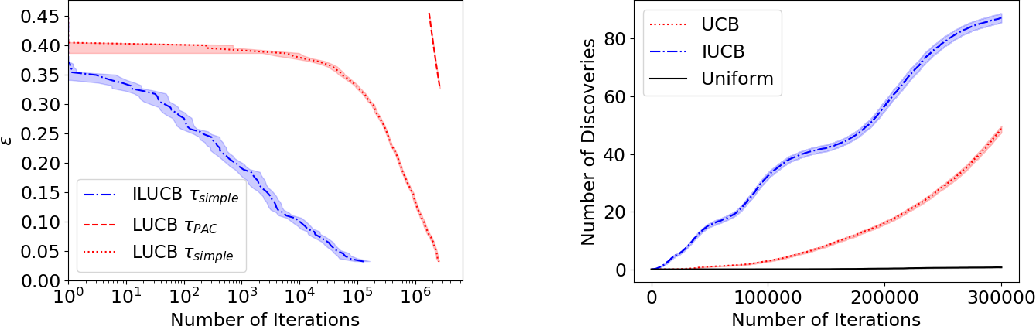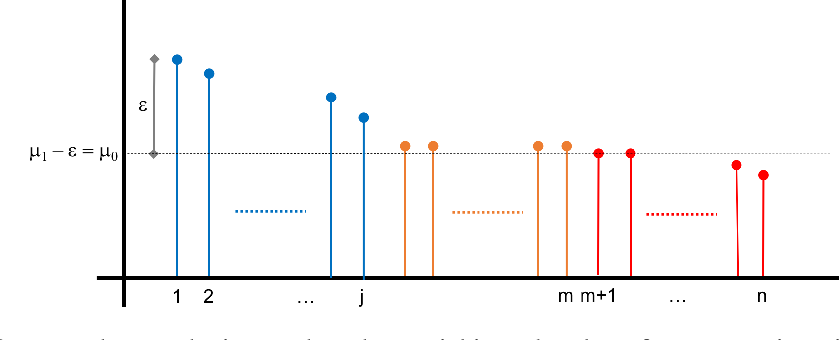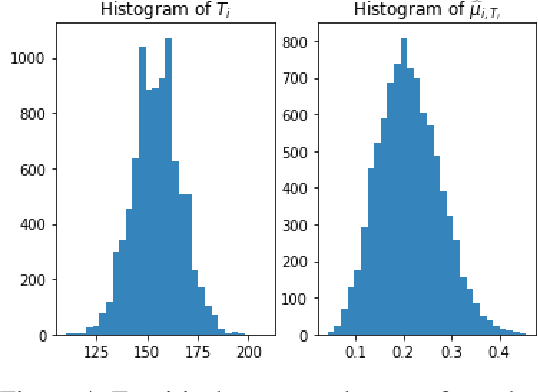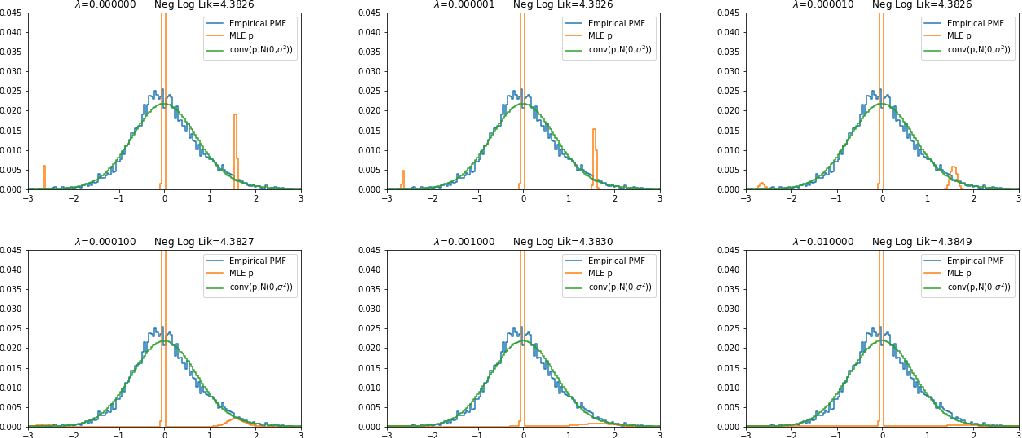We consider two multi-armed bandit problems with $n$ arms: (i) given an $\epsilon > 0$, identify an arm with mean that is within $\epsilon$ of the largest mean and (ii) given a threshold $\mu_0$ and integer $k$, identify $k$ arms with means larger than $\mu_0$. Existing lower bounds and algorithms for the PAC framework suggest that both of these problems require $\Omega(n)$ samples. However, we argue that these definitions not only conflict with how these algorithms are used in practice, but also that these results disagree with intuition that says (i) requires only $\Theta(\frac{n}{m})$ samples where $m = |\{ i : \mu_i > \max_{i \in [n]} \mu_i - \epsilon\}|$ and (ii) requires $\Theta(\frac{n}{m}k)$ samples where $m = |\{ i : \mu_i > \mu_0 \}|$. We provide definitions that formalize these intuitions, obtain lower bounds that match the above sample complexities, and develop explicit, practical algorithms that achieve nearly matching upper bounds.

Click to Read Paper and Get Code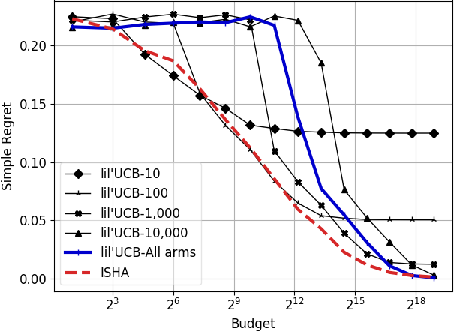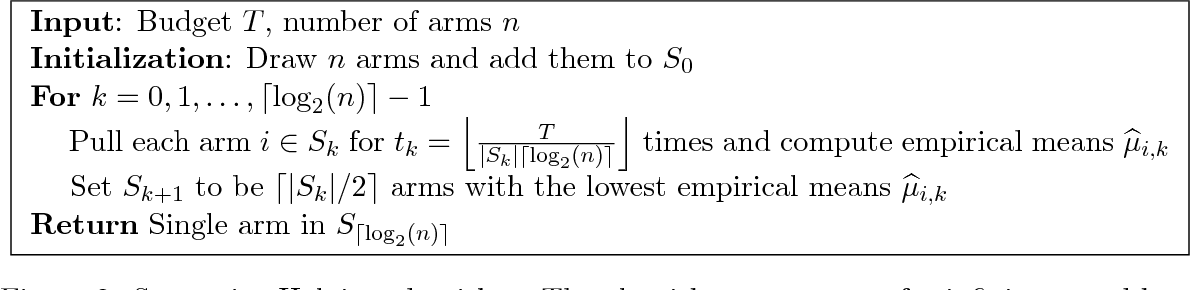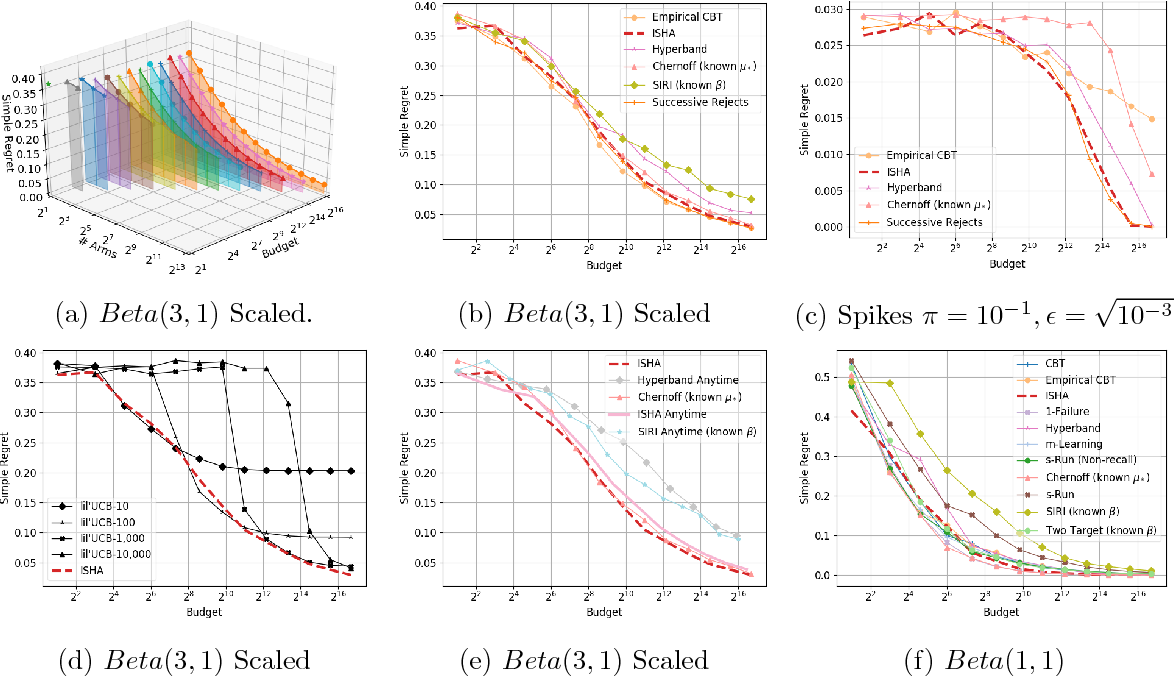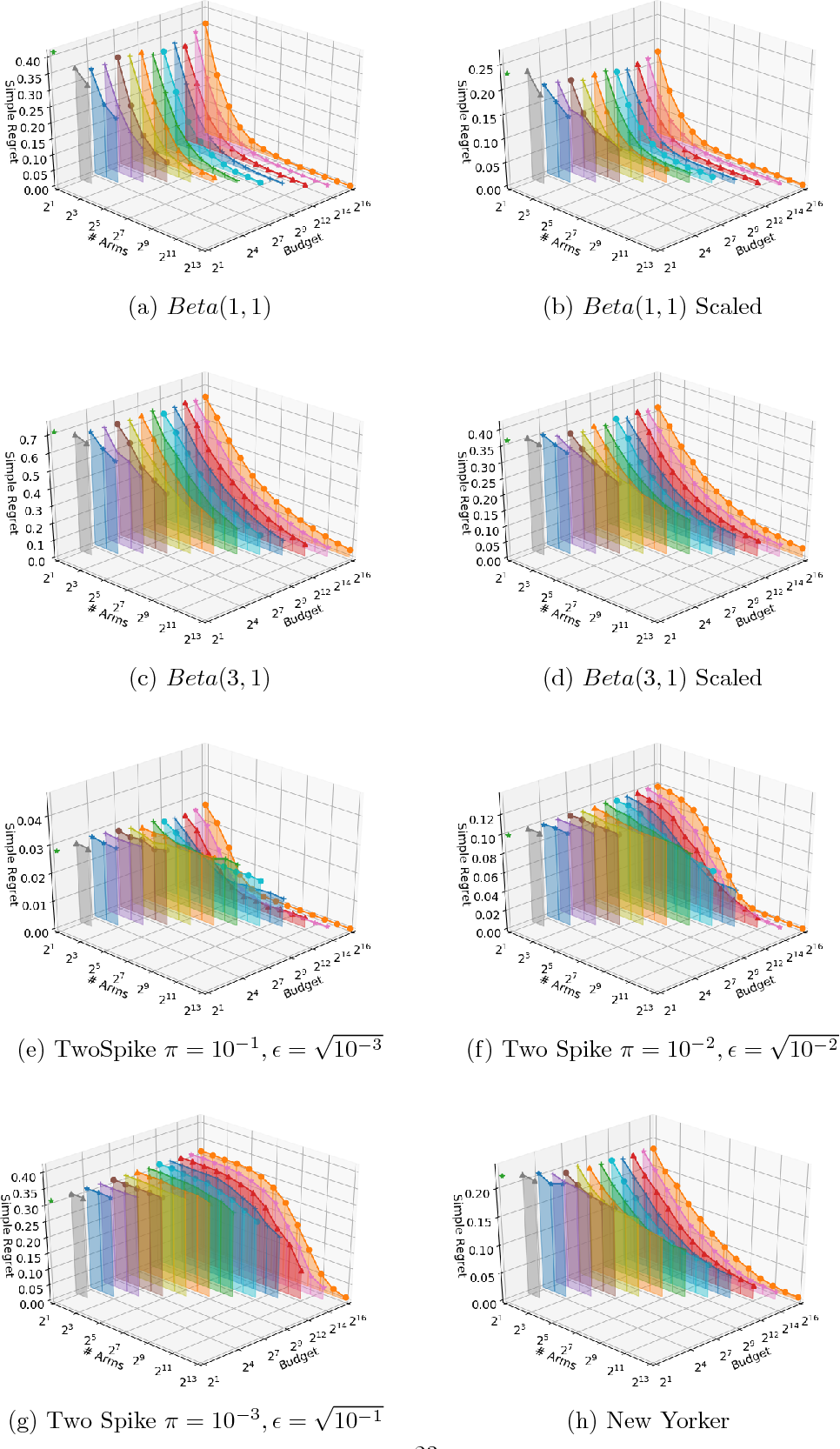This paper considers a multi-armed bandit game where the number of arms is much larger than the maximum budget and is effectively infinite. We characterize necessary and sufficient conditions on the total budget for an algorithm to return an {\epsilon}-good arm with probability at least 1 - {\delta}. In such situations, the sample complexity depends on {\epsilon}, {\delta} and the so-called reservoir distribution {\nu} from which the means of the arms are drawn iid. While a substantial literature has developed around analyzing specific cases of {\nu} such as the beta distribution, our analysis makes no assumption about the form of {\nu}. Our algorithm is based on successive halving with the surprising exception that arms start to be discarded after just a single pull, requiring an analysis that goes beyond concentration alone. The provable correctness of this algorithm also provides an explanation for the empirical observation that the most aggressive bracket of the Hyperband algorithm of Li et al. (2017) for hyperparameter tuning is almost always best.

Click to Read Paper and Get Code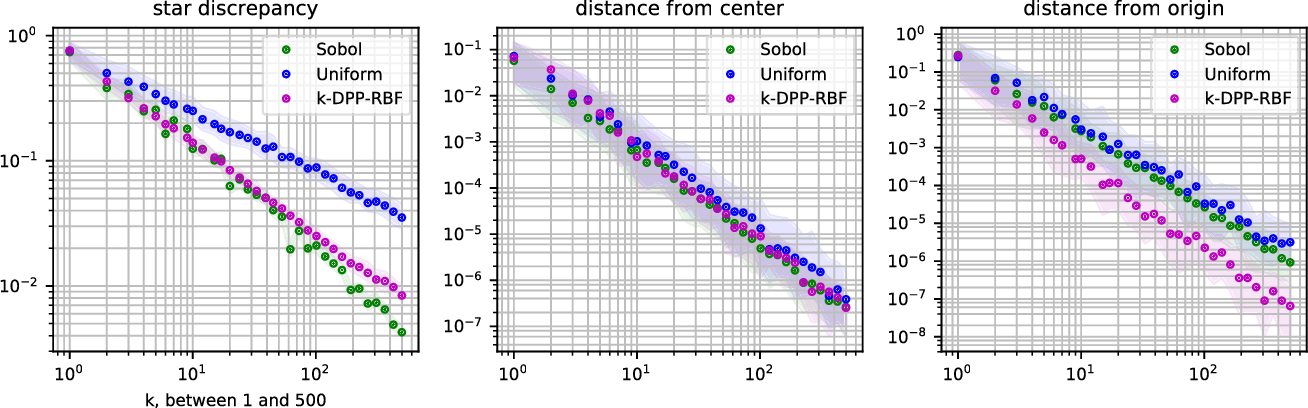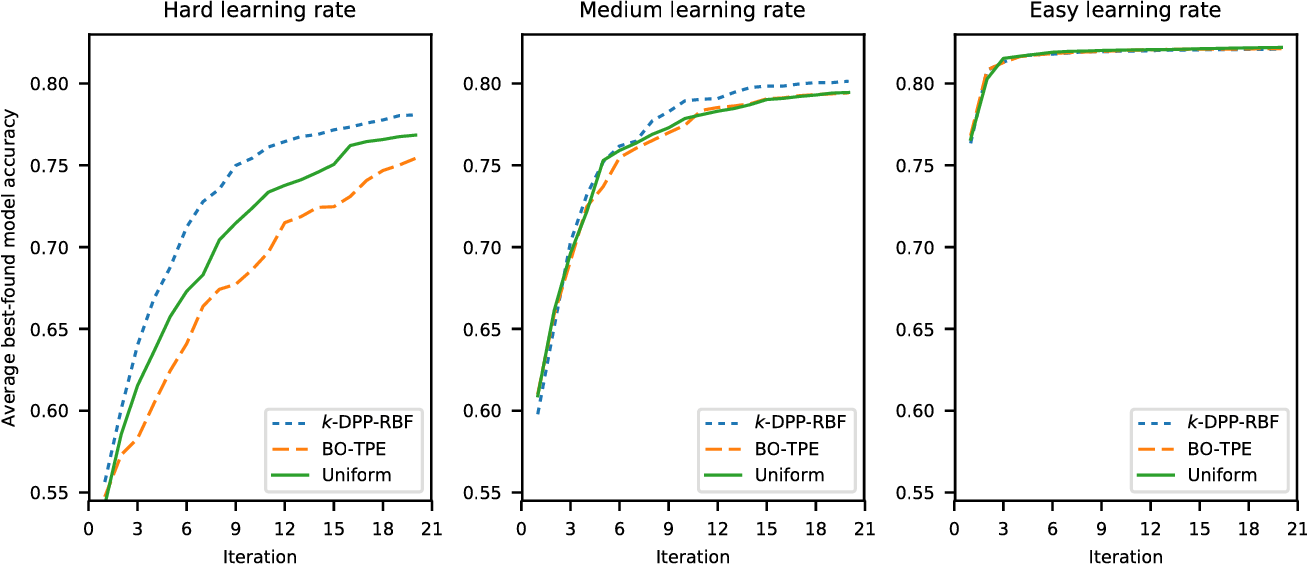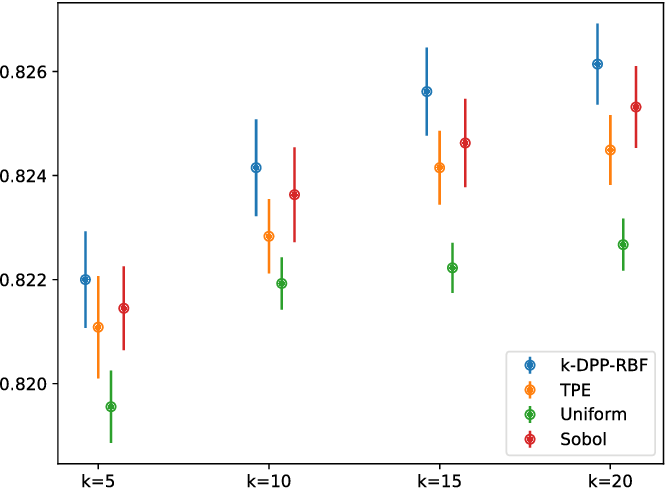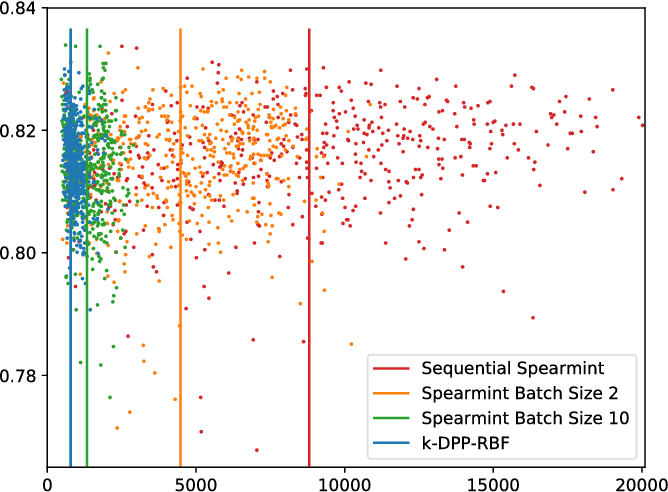Driven by the need for parallelizable hyperparameter optimization methods, this paper studies \emph{open loop} search methods: sequences that are predetermined and can be generated before a single configuration is evaluated. Examples include grid search, uniform random search, low discrepancy sequences, and other sampling distributions. In particular, we propose the use of $k$-determinantal point processes in hyperparameter optimization via random search. Compared to conventional uniform random search where hyperparameter settings are sampled independently, a $k$-DPP promotes diversity. We describe an approach that transforms hyperparameter search spaces for efficient use with a $k$-DPP. In addition, we introduce a novel Metropolis-Hastings algorithm which can sample from $k$-DPPs defined over any space from which uniform samples can be drawn, including spaces with a mixture of discrete and continuous dimensions or tree structure. Our experiments show significant benefits in realistic scenarios with a limited budget for training supervised learners, whether in serial or parallel.

Click to Read Paper and Get Code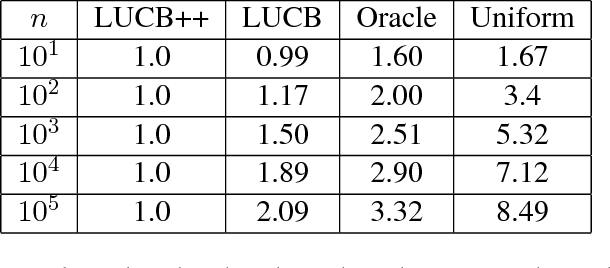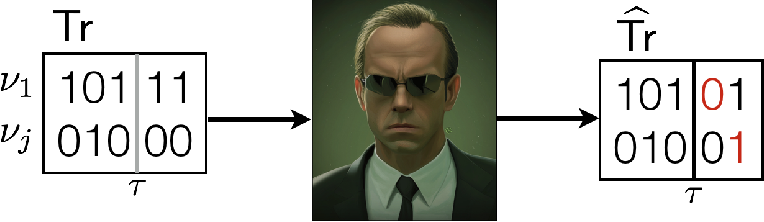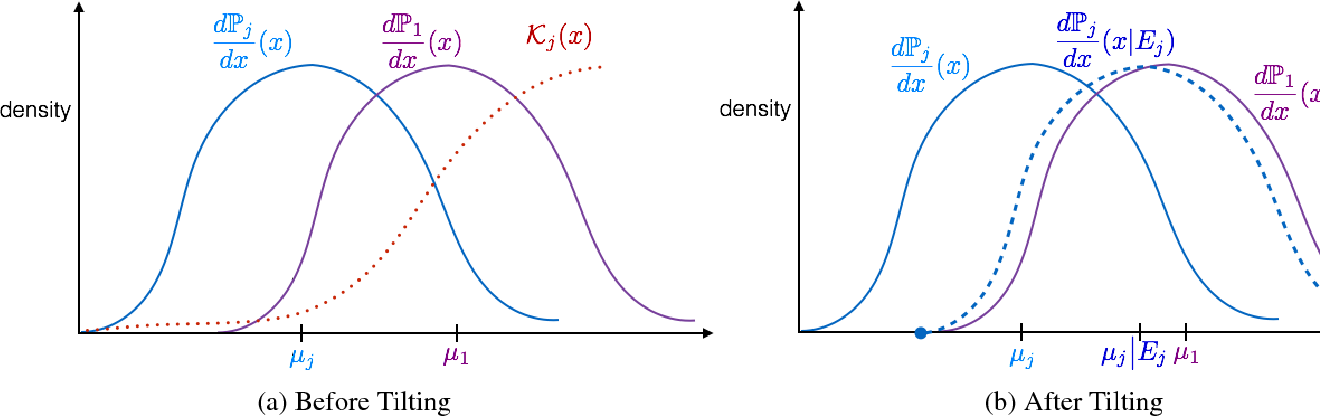We propose a novel technique for analyzing adaptive sampling called the {\em Simulator}. Our approach differs from the existing methods by considering not how much information could be gathered by any fixed sampling strategy, but how difficult it is to distinguish a good sampling strategy from a bad one given the limited amount of data collected up to any given time. This change of perspective allows us to match the strength of both Fano and change-of-measure techniques, without succumbing to the limitations of either method. For concreteness, we apply our techniques to a structured multi-arm bandit problem in the fixed-confidence pure exploration setting, where we show that the constraints on the means imply a substantial gap between the moderate-confidence sample complexity, and the asymptotic sample complexity as $\delta \to 0$ found in the literature. We also prove the first instance-based lower bounds for the top-k problem which incorporate the appropriate log-factors. Moreover, our lower bounds zero-in on the number of times each \emph{individual} arm needs to be pulled, uncovering new phenomena which are drowned out in the aggregate sample complexity. Our new analysis inspires a simple and near-optimal algorithm for the best-arm and top-k identification, the first {\em practical} algorithm of its kind for the latter problem which removes extraneous log factors, and outperforms the state-of-the-art in experiments.

Click to Read Paper and Get Code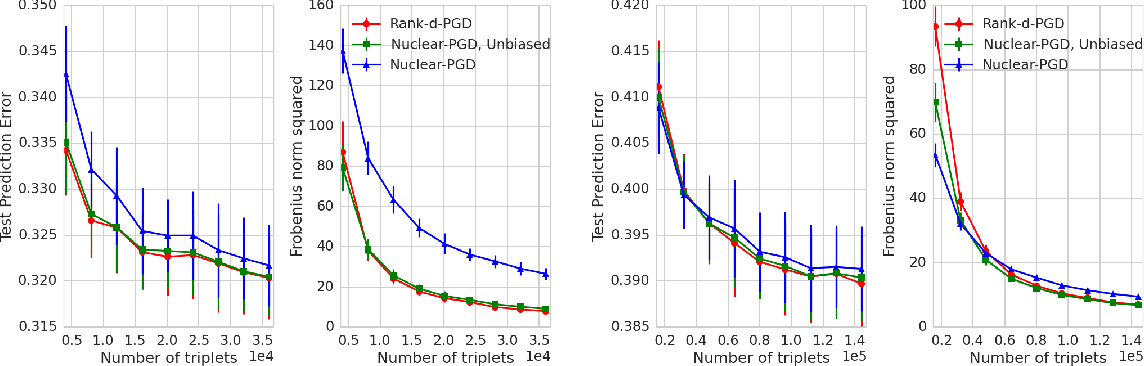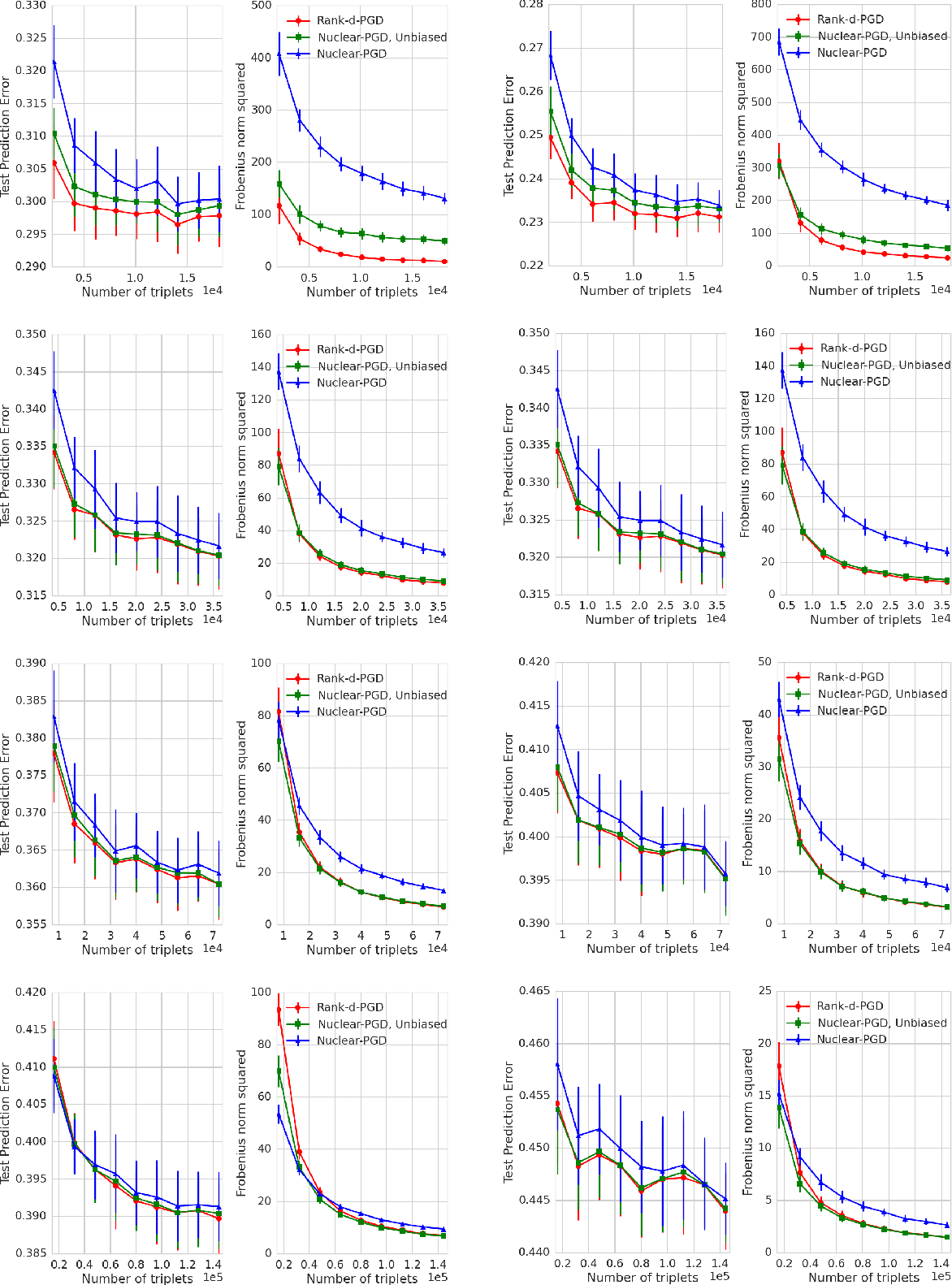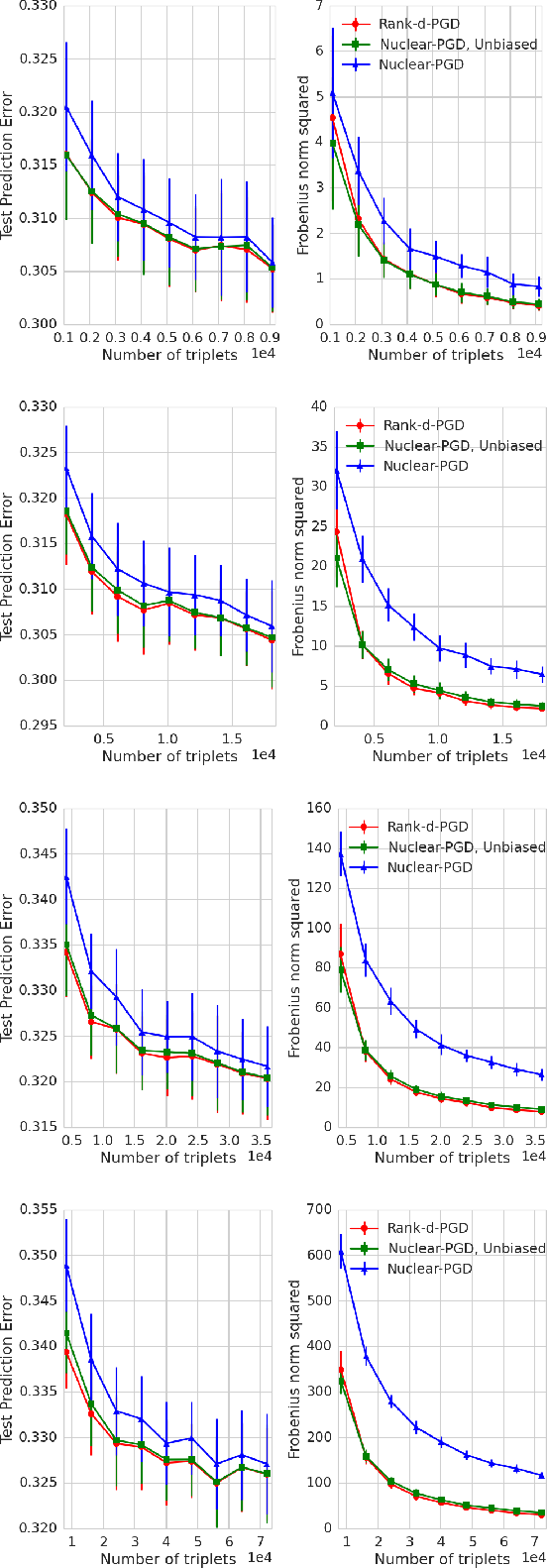The goal of ordinal embedding is to represent items as points in a low-dimensional Euclidean space given a set of constraints in the form of distance comparisons like "item $i$ is closer to item $j$ than item $k$". Ordinal constraints like this often come from human judgments. To account for errors and variation in judgments, we consider the noisy situation in which the given constraints are independently corrupted by reversing the correct constraint with some probability. This paper makes several new contributions to this problem. First, we derive prediction error bounds for ordinal embedding with noise by exploiting the fact that the rank of a distance matrix of points in $\mathbb{R}^d$ is at most $d+2$. These bounds characterize how well a learned embedding predicts new comparative judgments. Second, we investigate the special case of a known noise model and study the Maximum Likelihood estimator. Third, knowledge of the noise model enables us to relate prediction errors to embedding accuracy. This relationship is highly non-trivial since we show that the linear map corresponding to distance comparisons is non-invertible, but there exists a nonlinear map that is invertible. Fourth, two new algorithms for ordinal embedding are proposed and evaluated in experiments.

Click to Read Paper and Get Code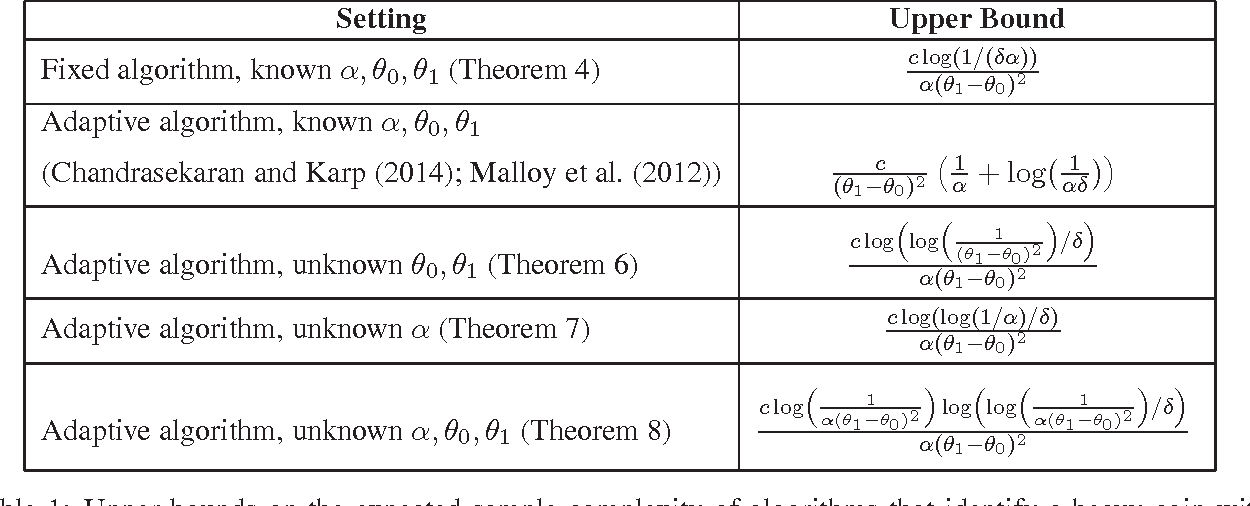This paper studies the trade-off between two different kinds of pure exploration: breadth versus depth. The most biased coin problem asks how many total coin flips are required to identify a "heavy" coin from an infinite bag containing both "heavy" coins with mean $\theta_1 \in (0,1)$, and "light" coins with mean $\theta_0 \in (0,\theta_1)$, where heavy coins are drawn from the bag with probability $\alpha \in (0,1/2)$. The key difficulty of this problem lies in distinguishing whether the two kinds of coins have very similar means, or whether heavy coins are just extremely rare. This problem has applications in crowdsourcing, anomaly detection, and radio spectrum search. Chandrasekaran et. al. (2014) recently introduced a solution to this problem but it required perfect knowledge of $\theta_0,\theta_1,\alpha$. In contrast, we derive algorithms that are adaptive to partial or absent knowledge of the problem parameters. Moreover, our techniques generalize beyond coins to more general instances of infinitely many armed bandit problems. We also prove lower bounds that show our algorithm's upper bounds are tight up to $\log$ factors, and on the way characterize the sample complexity of differentiating between a single parametric distribution and a mixture of two such distributions. As a result, these bounds have surprising implications both for solutions to the most biased coin problem and for anomaly detection when only partial information about the parameters is known.

Click to Read Paper and Get Code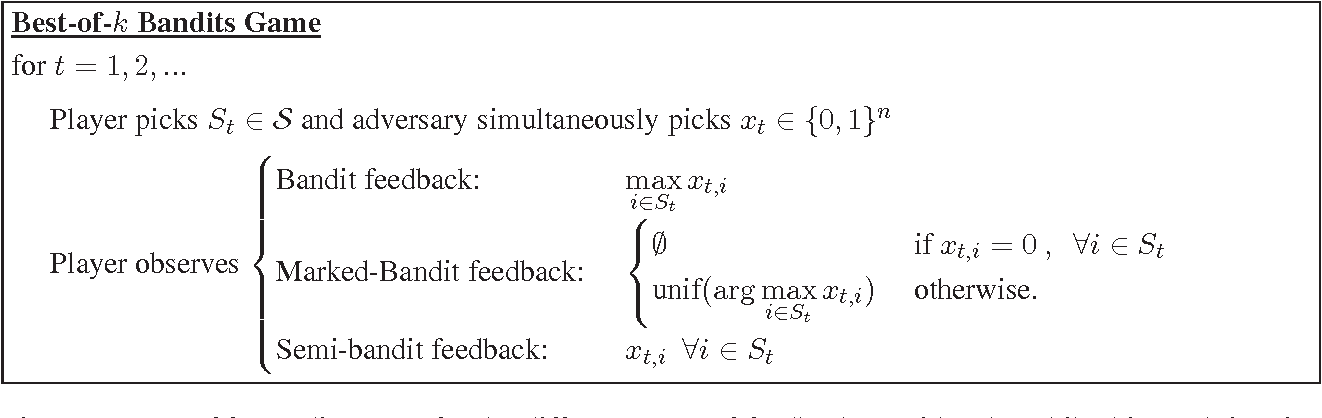This paper studies the Best-of-K Bandit game: At each time the player chooses a subset S among all N-choose-K possible options and observes reward max(X(i) : i in S) where X is a random vector drawn from a joint distribution. The objective is to identify the subset that achieves the highest expected reward with high probability using as few queries as possible. We present distribution-dependent lower bounds based on a particular construction which force a learner to consider all N-choose-K subsets, and match naive extensions of known upper bounds in the bandit setting obtained by treating each subset as a separate arm. Nevertheless, we present evidence that exhaustive search may be avoided for certain, favorable distributions because the influence of high-order order correlations may be dominated by lower order statistics. Finally, we present an algorithm and analysis for independent arms, which mitigates the surprising non-trivial information occlusion that occurs due to only observing the max in the subset. This may inform strategies for more general dependent measures, and we complement these result with independent-arm lower bounds.

Click to Read Paper and Get Code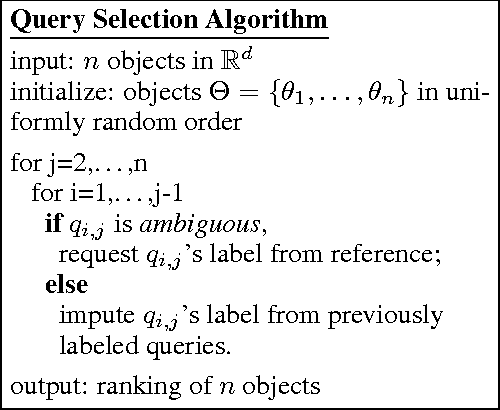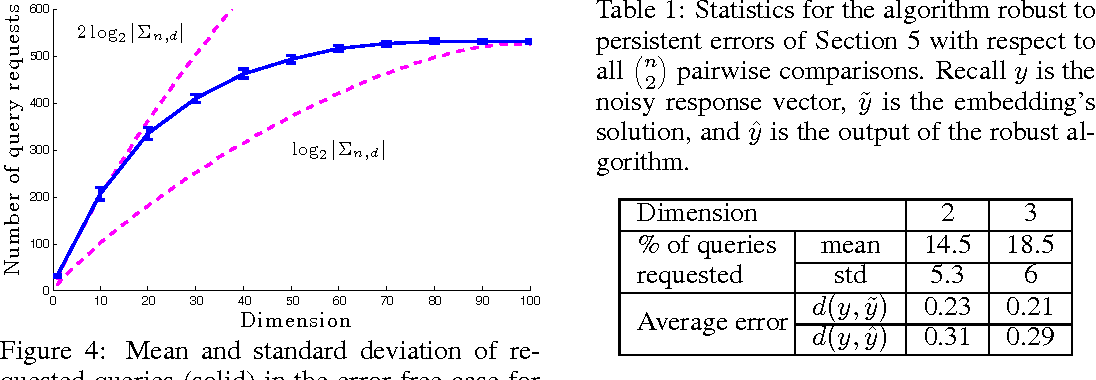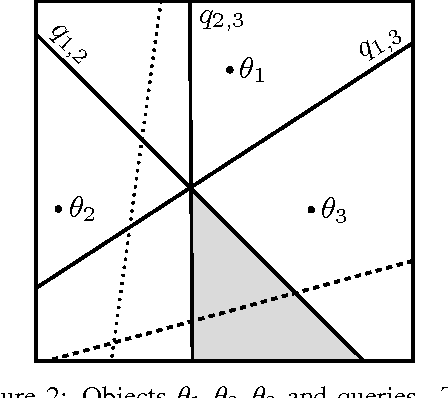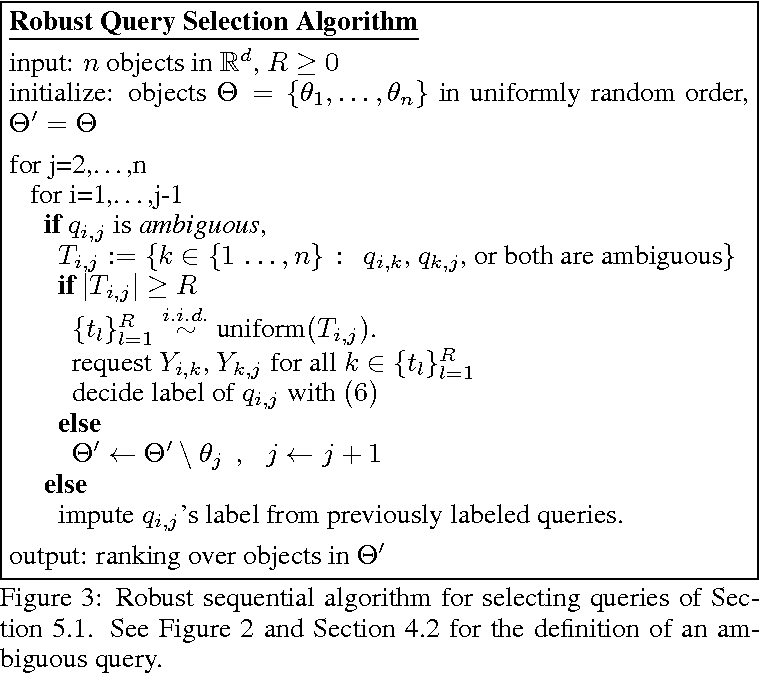This paper examines the problem of ranking a collection of objects using pairwise comparisons (rankings of two objects). In general, the ranking of $n$ objects can be identified by standard sorting methods using $n log_2 n$ pairwise comparisons. We are interested in natural situations in which relationships among the objects may allow for ranking using far fewer pairwise comparisons. Specifically, we assume that the objects can be embedded into a $d$-dimensional Euclidean space and that the rankings reflect their relative distances from a common reference point in $R^d$. We show that under this assumption the number of possible rankings grows like $n^{2d}$ and demonstrate an algorithm that can identify a randomly selected ranking using just slightly more than $d log n$ adaptively selected pairwise comparisons, on average. If instead the comparisons are chosen at random, then almost all pairwise comparisons must be made in order to identify any ranking. In addition, we propose a robust, error-tolerant algorithm that only requires that the pairwise comparisons are probably correct. Experimental studies with synthetic and real datasets support the conclusions of our theoretical analysis.

* 17 pages, an extended version of our NIPS 2011 paper. The new version revises the argument of the robust section and slightly modifies the result there to give it more impact
Click to Read Paper and Get Code
In this paper we introduce the transductive linear bandit problem: given a set of measurement vectors $\mathcal{X}\subset \mathbb{R}^d$, a set of items $\mathcal{Z}\subset \mathbb{R}^d$, a fixed confidence $\delta$, and an unknown vector $\theta^{\ast}\in \mathbb{R}^d$, the goal is to infer $\text{argmax}_{z\in \mathcal{Z}} z^\top\theta^\ast$ with probability $1-\delta$ by making as few sequentially chosen noisy measurements of the form $x^\top\theta^{\ast}$ as possible. When $\mathcal{X}=\mathcal{Z}$, this setting generalizes linear bandits, and when $\mathcal{X}$ is the standard basis vectors and $\mathcal{Z}\subset \{0,1\}^d$, combinatorial bandits. Such a transductive setting naturally arises when the set of measurement vectors is limited due to factors such as availability or cost. As an example, in drug discovery the compounds and dosages $\mathcal{X}$ a practitioner may be willing to evaluate in the lab in vitro due to cost or safety reasons may differ vastly from those compounds and dosages $\mathcal{Z}$ that can be safely administered to patients in vivo. Alternatively, in recommender systems for books, the set of books $\mathcal{X}$ a user is queried about may be restricted to well known best-sellers even though the goal might be to recommend more esoteric titles $\mathcal{Z}$. In this paper, we provide instance-dependent lower bounds for the transductive setting, an algorithm that matches these up to logarithmic factors, and an evaluation. In particular, we provide the first non-asymptotic algorithm for linear bandits that nearly achieves the information theoretic lower bound.

Click to Read Paper and Get Code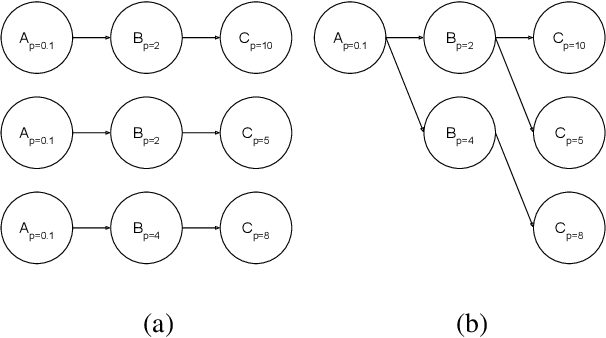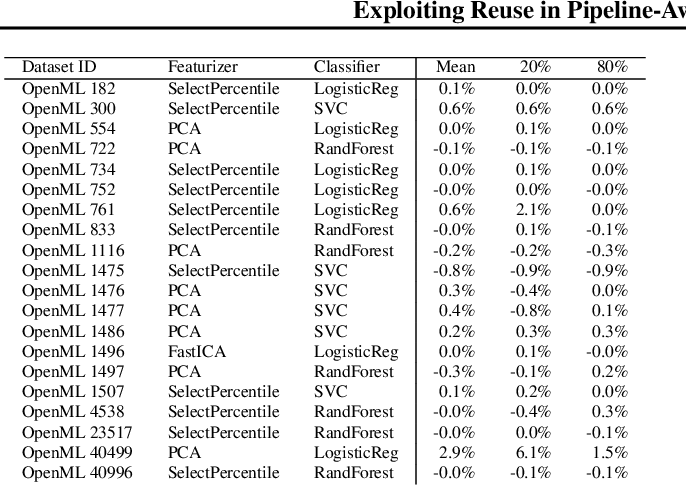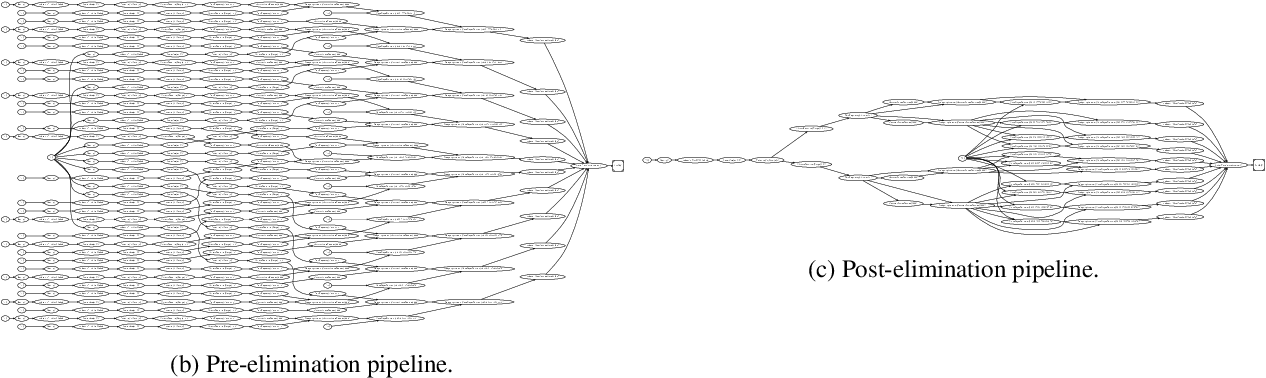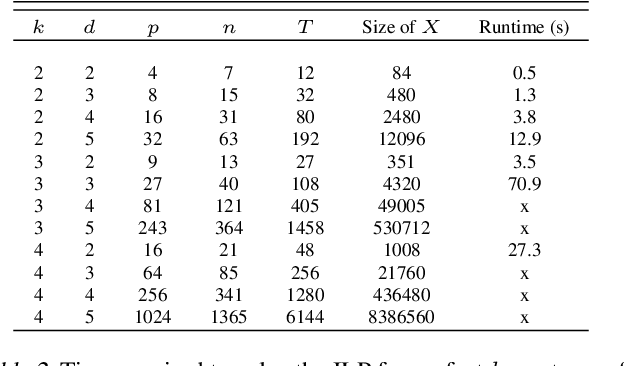Hyperparameter tuning of multi-stage pipelines introduces a significant computational burden. Motivated by the observation that work can be reused across pipelines if the intermediate computations are the same, we propose a pipeline-aware approach to hyperparameter tuning. Our approach optimizes both the design and execution of pipelines to maximize reuse. We design pipelines amenable for reuse by (i) introducing a novel hybrid hyperparameter tuning method called gridded random search, and (ii) reducing the average training time in pipelines by adapting early-stopping hyperparameter tuning approaches. We then realize the potential for reuse during execution by introducing a novel caching problem for ML workloads which we pose as a mixed integer linear program (ILP), and subsequently evaluating various caching heuristics relative to the optimal solution of the ILP. We conduct experiments on simulated and real-world machine learning pipelines to show that a pipeline-aware approach to hyperparameter tuning can offer over an order-of-magnitude speedup over independently evaluating pipeline configurations.

Click to Read Paper and Get Code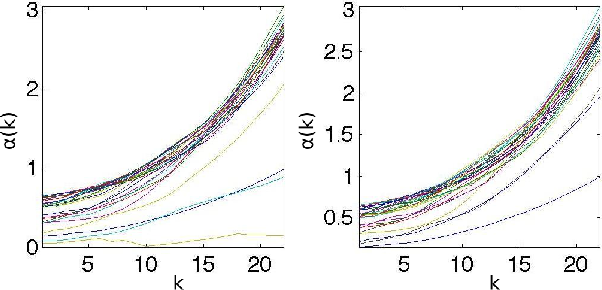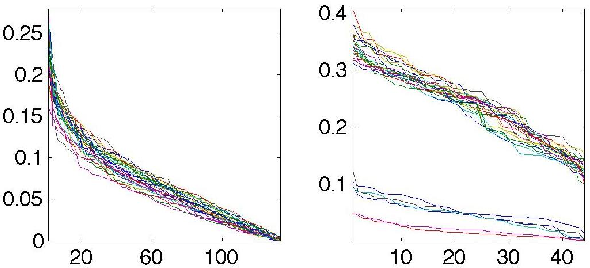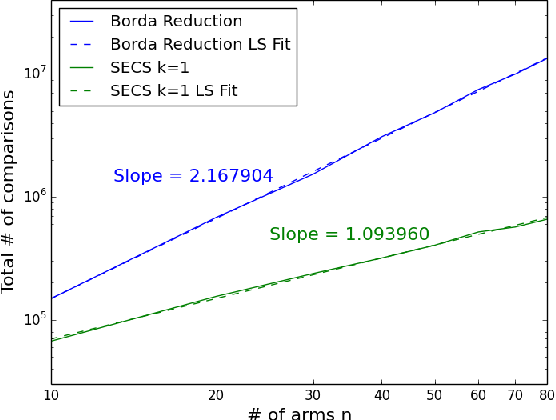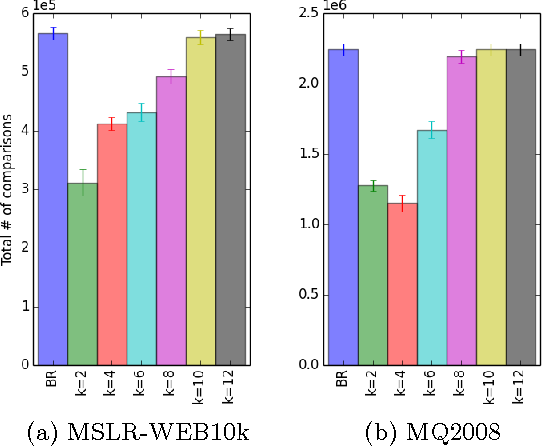The dueling bandit problem is a variation of the classical multi-armed bandit in which the allowable actions are noisy comparisons between pairs of arms. This paper focuses on a new approach for finding the "best" arm according to the Borda criterion using noisy comparisons. We prove that in the absence of structural assumptions, the sample complexity of this problem is proportional to the sum of the inverse squared gaps between the Borda scores of each suboptimal arm and the best arm. We explore this dependence further and consider structural constraints on the pairwise comparison matrix (a particular form of sparsity natural to this problem) that can significantly reduce the sample complexity. This motivates a new algorithm called Successive Elimination with Comparison Sparsity (SECS) that exploits sparsity to find the Borda winner using fewer samples than standard algorithms. We also evaluate the new algorithm experimentally with synthetic and real data. The results show that the sparsity model and the new algorithm can provide significant improvements over standard approaches.

Click to Read Paper and Get Code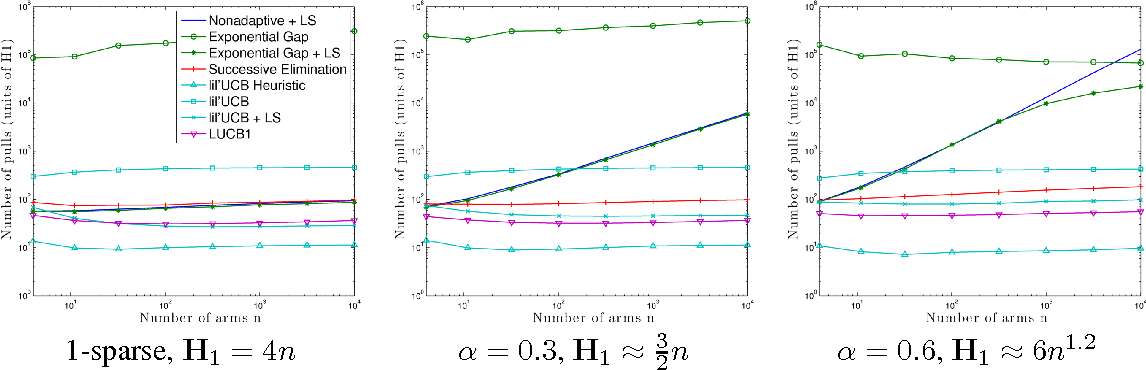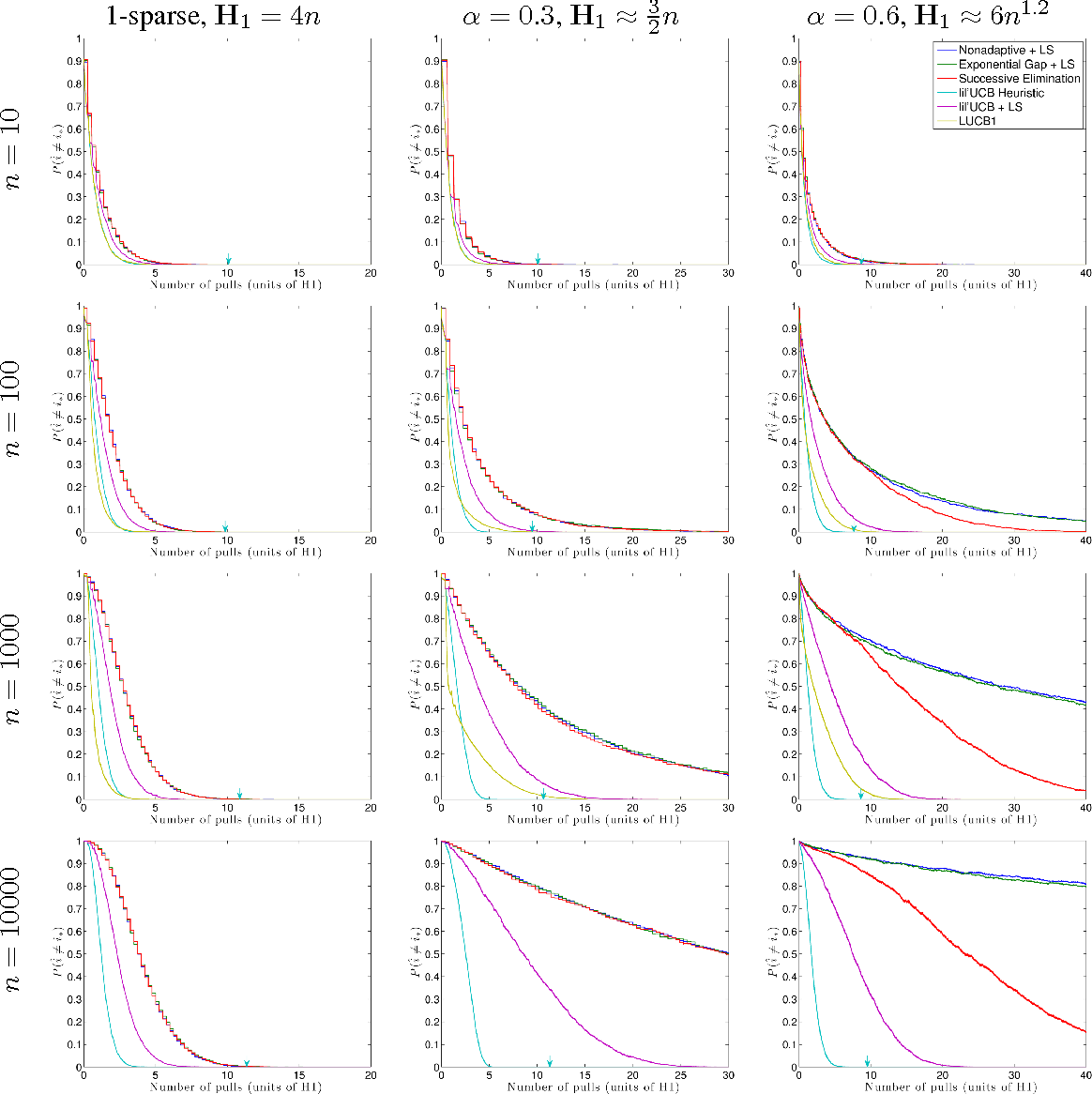The paper proposes a novel upper confidence bound (UCB) procedure for identifying the arm with the largest mean in a multi-armed bandit game in the fixed confidence setting using a small number of total samples. The procedure cannot be improved in the sense that the number of samples required to identify the best arm is within a constant factor of a lower bound based on the law of the iterated logarithm (LIL). Inspired by the LIL, we construct our confidence bounds to explicitly account for the infinite time horizon of the algorithm. In addition, by using a novel stopping time for the algorithm we avoid a union bound over the arms that has been observed in other UCB-type algorithms. We prove that the algorithm is optimal up to constants and also show through simulations that it provides superior performance with respect to the state-of-the-art.

Click to Read Paper and Get Code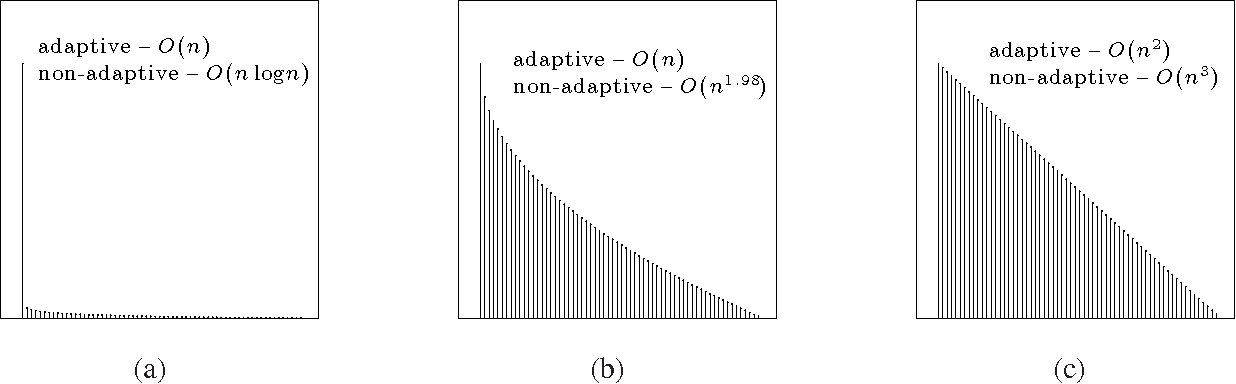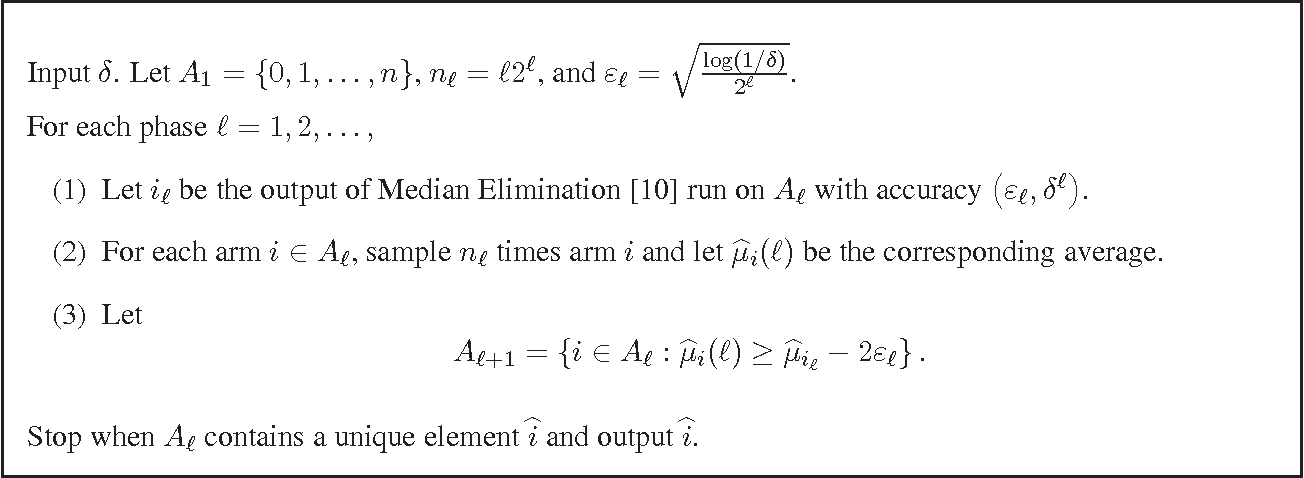Sampling from distributions to find the one with the largest mean arises in a broad range of applications, and it can be mathematically modeled as a multi-armed bandit problem in which each distribution is associated with an arm. This paper studies the sample complexity of identifying the best arm (largest mean) in a multi-armed bandit problem. Motivated by large-scale applications, we are especially interested in identifying situations where the total number of samples that are necessary and sufficient to find the best arm scale linearly with the number of arms. We present a single-parameter multi-armed bandit model that spans the range from linear to superlinear sample complexity. We also give a new algorithm for best arm identification, called PRISM, with linear sample complexity for a wide range of mean distributions. The algorithm, like most exploration procedures for multi-armed bandits, is adaptive in the sense that the next arms to sample are selected based on previous samples. We compare the sample complexity of adaptive procedures with simpler non-adaptive procedures using new lower bounds. For many problem instances, the increased sample complexity required by non-adaptive procedures is a polynomial factor of the number of arms.

Click to Read Paper and Get Code
This paper provides lower bounds on the convergence rate of Derivative Free Optimization (DFO) with noisy function evaluations, exposing a fundamental and unavoidable gap between the performance of algorithms with access to gradients and those with access to only function evaluations. However, there are situations in which DFO is unavoidable, and for such situations we propose a new DFO algorithm that is proved to be near optimal for the class of strongly convex objective functions. A distinctive feature of the algorithm is that it uses only Boolean-valued function comparisons, rather than function evaluations. This makes the algorithm useful in an even wider range of applications, such as optimization based on paired comparisons from human subjects, for example. We also show that regardless of whether DFO is based on noisy function evaluations or Boolean-valued function comparisons, the convergence rate is the same.

Click to Read Paper and Get Code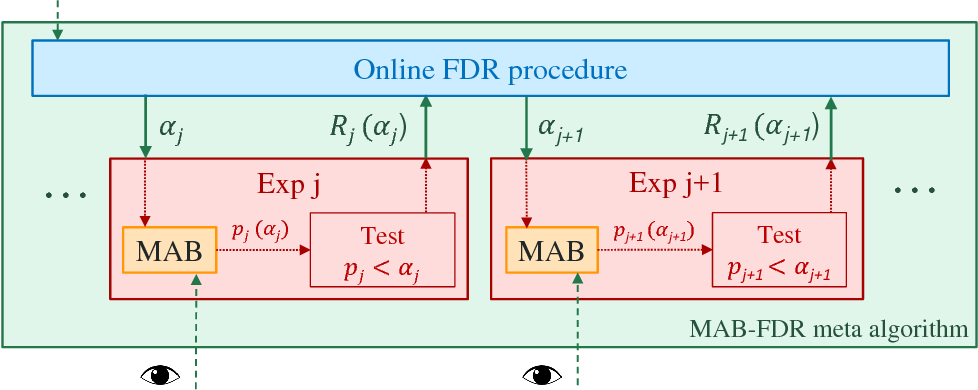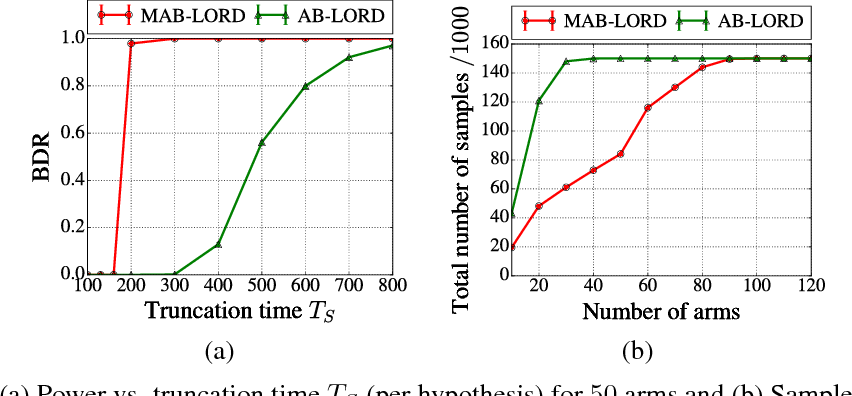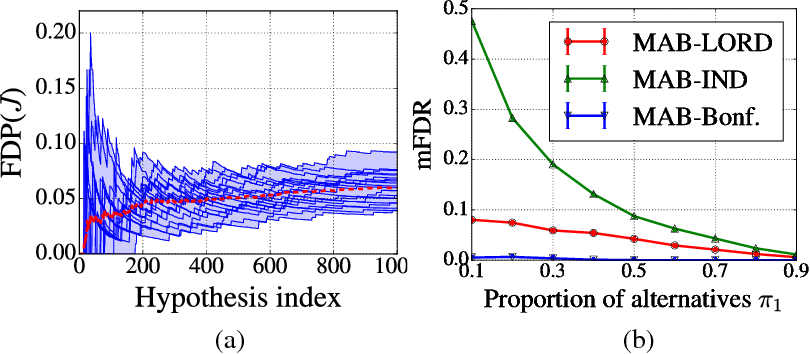We propose an alternative framework to existing setups for controlling false alarms when multiple A/B tests are run over time. This setup arises in many practical applications, e.g. when pharmaceutical companies test new treatment options against control pills for different diseases, or when internet companies test their default webpages versus various alternatives over time. Our framework proposes to replace a sequence of A/B tests by a sequence of best-arm MAB instances, which can be continuously monitored by the data scientist. When interleaving the MAB tests with an an online false discovery rate (FDR) algorithm, we can obtain the best of both worlds: low sample complexity and any time online FDR control. Our main contributions are: (i) to propose reasonable definitions of a null hypothesis for MAB instances; (ii) to demonstrate how one can derive an always-valid sequential p-value that allows continuous monitoring of each MAB test; and (iii) to show that using rejection thresholds of online-FDR algorithms as the confidence levels for the MAB algorithms results in both sample-optimality, high power and low FDR at any point in time. We run extensive simulations to verify our claims, and also report results on real data collected from the New Yorker Cartoon Caption contest.

* Published as a conference paper at NIPS 2017
Click to Read Paper and Get Code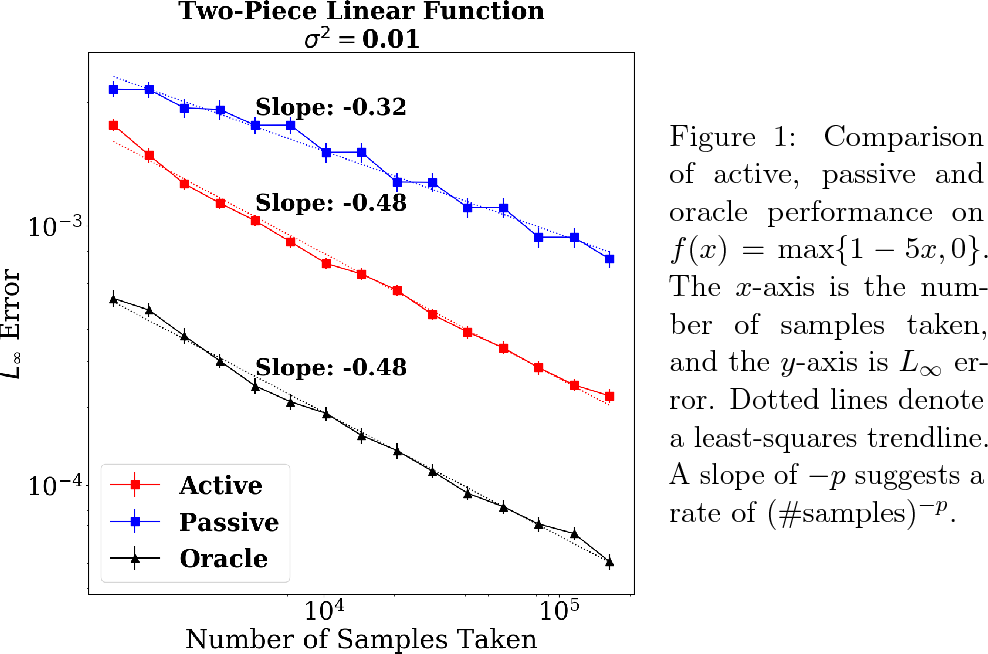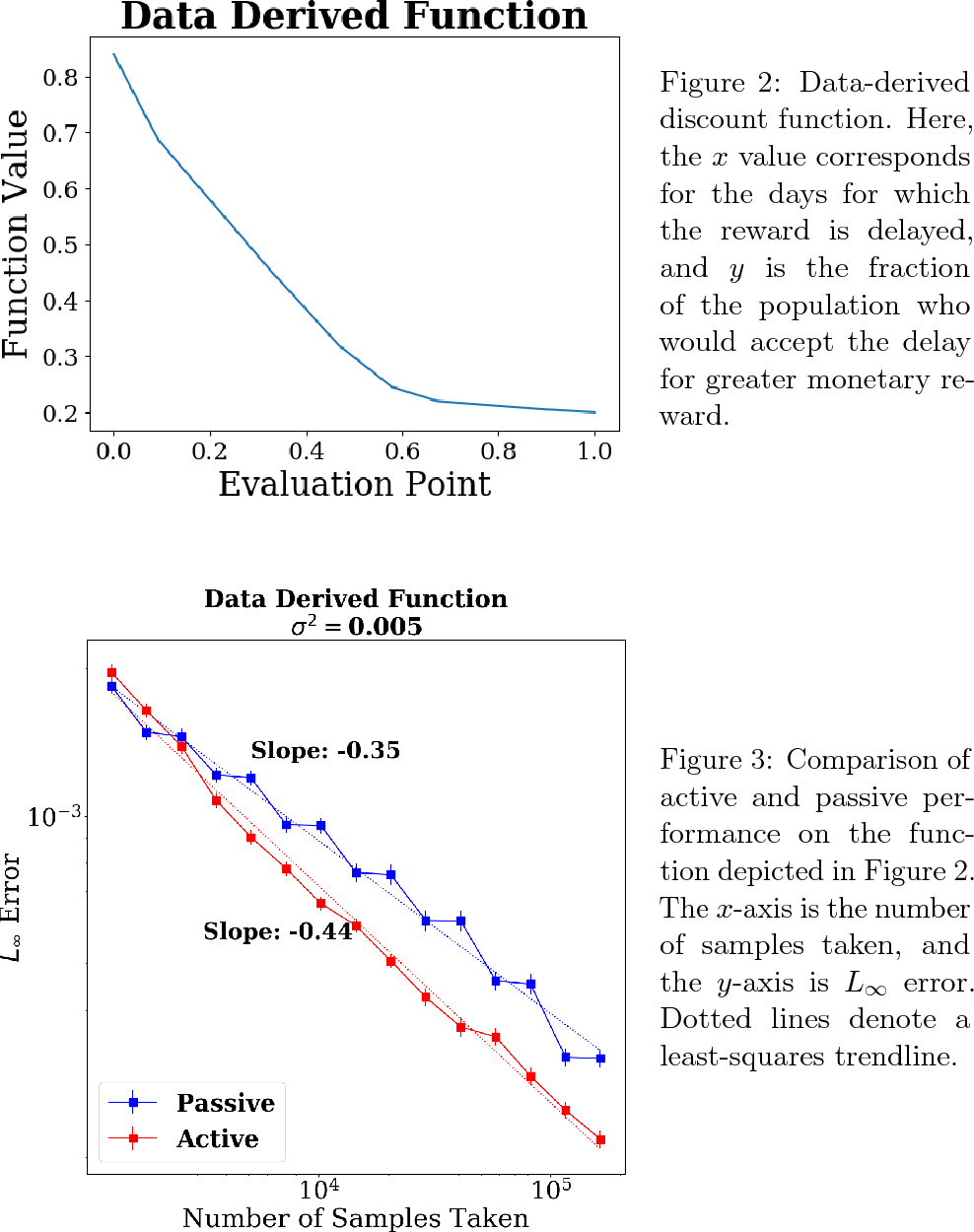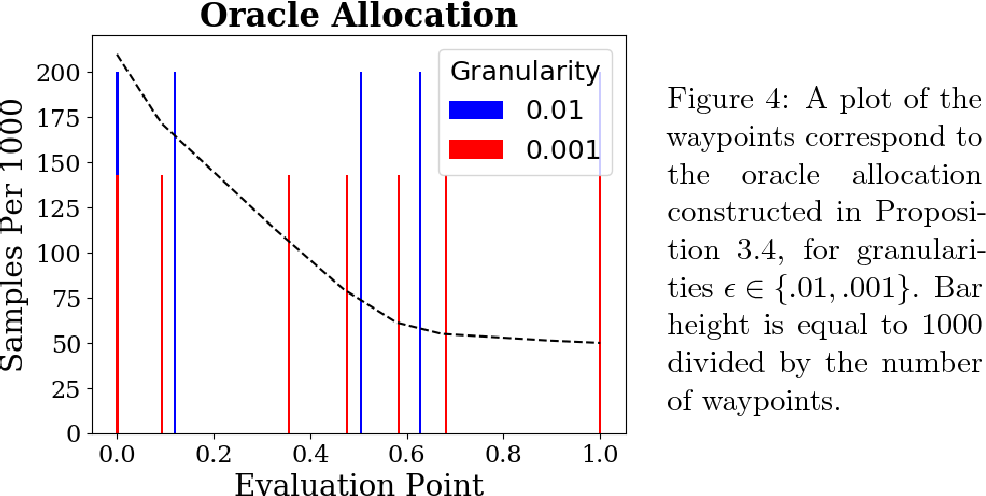In this paper, we introduce the first principled adaptive-sampling procedure for learning a convex function in the $L_\infty$ norm, a problem that arises often in the behavioral and social sciences. We present a function-specific measure of complexity and use it to prove that, for each convex function $f_{\star}$, our algorithm nearly attains the information-theoretically optimal, function-specific error rate. We also corroborate our theoretical contributions with numerical experiments, finding that our method substantially outperforms passive, uniform sampling for favorable synthetic and data-derived functions in low-noise settings with large sampling budgets. Our results also suggest an idealized "oracle strategy", which we use to gauge the potential advance of any adaptive-sampling strategy over passive sampling, for any given convex function.

Click to Read Paper and Get Code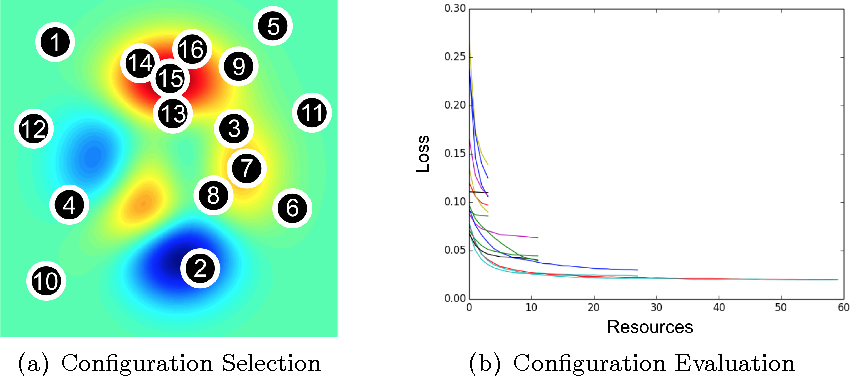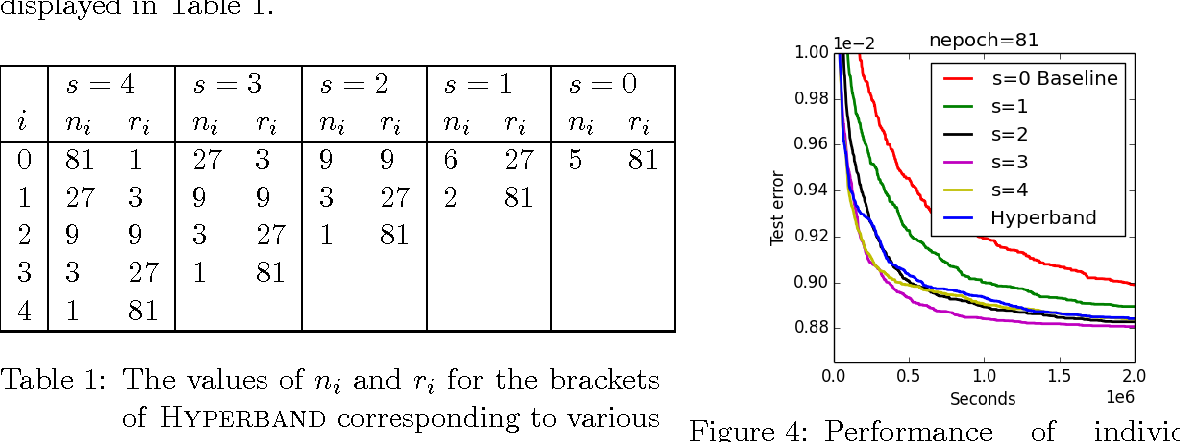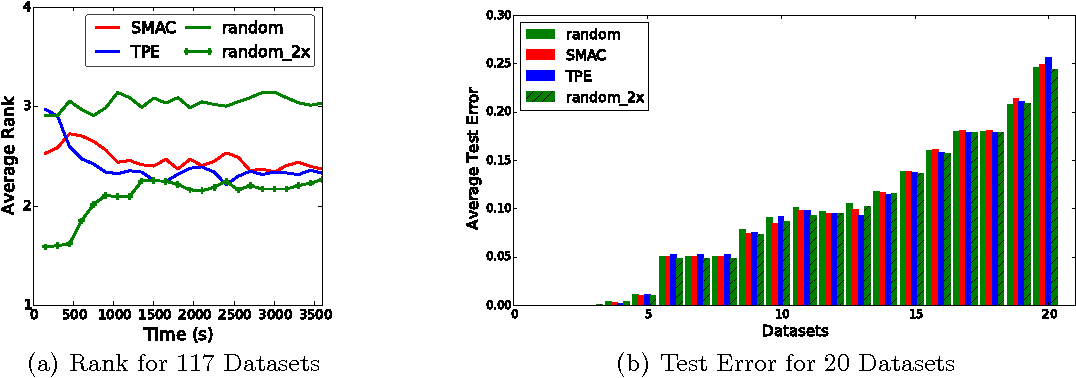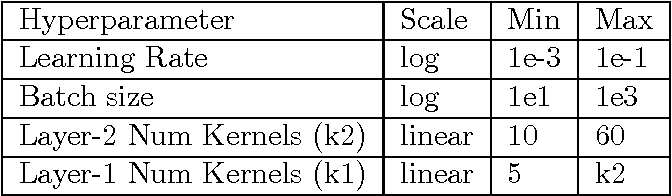Performance of machine learning algorithms depends critically on identifying a good set of hyperparameters. While recent approaches use Bayesian optimization to adaptively select configurations, we focus on speeding up random search through adaptive resource allocation and early-stopping. We formulate hyperparameter optimization as a pure-exploration non-stochastic infinite-armed bandit problem where a predefined resource like iterations, data samples, or features is allocated to randomly sampled configurations. We introduce a novel algorithm, Hyperband, for this framework and analyze its theoretical properties, providing several desirable guarantees. Furthermore, we compare Hyperband with popular Bayesian optimization methods on a suite of hyperparameter optimization problems. We observe that Hyperband can provide over an order-of-magnitude speedup over our competitor set on a variety of deep-learning and kernel-based learning problems.

* Journal of Machine Learning Research 18 (2018) 1-52
* Changes: - Updated to JMLR version
Click to Read Paper and Get Code